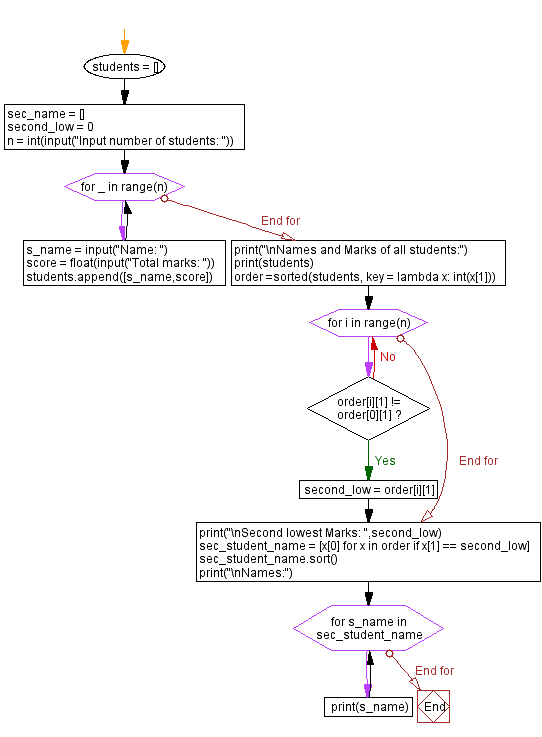﻿ Python: Find the second lowest total marks of any student(s) from the given names and total marks of each student using lists and Lambda - w3resource# Python: Find the second lowest total marks of any student(s) from the given names and total marks of each student using lists and Lambda

## Python Collections: Exercise-16 with Solution

Write a Python program to find the second lowest total marks of any student(s) from the given names and marks of each student using lists and lambda. Input number of students, names and grades of each student.

Sample Solution:

Python Code:

``````students = []
sec_name = []
second_low = 0
n = int(input("Input number of students: "))
for _ in range(n):
s_name = input("Name: ")
score = float(input("Total marks: "))
students.append([s_name,score])
print("\nNames and Marks of all students:")
print(students)
order =sorted(students, key = lambda x: int(x))
for i in range(n):
if order[i] != order:
second_low = order[i]
break
print("\nSecond lowest Marks: ",second_low)
sec_student_name = [x for x in order if x == second_low]
sec_student_name.sort()
print("\nNames:")
for s_name in sec_student_name:
print(s_name)
```
```

Sample Output:

```Input number of students:  3
Name:  Avik Das
Total marks:  89
Name:  ayan Roy
Total marks:  75
Name:  Sayan Dutta
Total marks:  93

Names and Marks of all students:
[['Avik Das ', 89.0], ['ayan Roy', 75.0], ['Sayan Dutta', 93.0]]

Second lowest Marks:  89.0

Names:
Avik Das
```

Flowchart:## Visualize Python code execution:

The following tool visualize what the computer is doing step-by-step as it executes the said program:

Python Code Editor:

Have another way to solve this solution? Contribute your code (and comments) through Disqus.

What is the difficulty level of this exercise?

Test your Programming skills with w3resource's quiz.

﻿

## Python: Tips of the Day

The Zip() Function:

```>>> students = ('John', 'Mary', 'Mike')
>>> ages = (15, 17, 16)
>>> scores = (90, 88, 82, 17, 14)
>>> for student, age, score in zip(students, ages, scores):
...     print(f'{student}, age: {age}, score: {score}')
...
John, age: 15, score: 90
Mary, age: 17, score: 88
Mike, age: 16, score: 82
>>> zipped = zip(students, ages, scores)
>>> a, b, c = zip(*zipped)
>>> print(b)
(15, 17, 16)
```